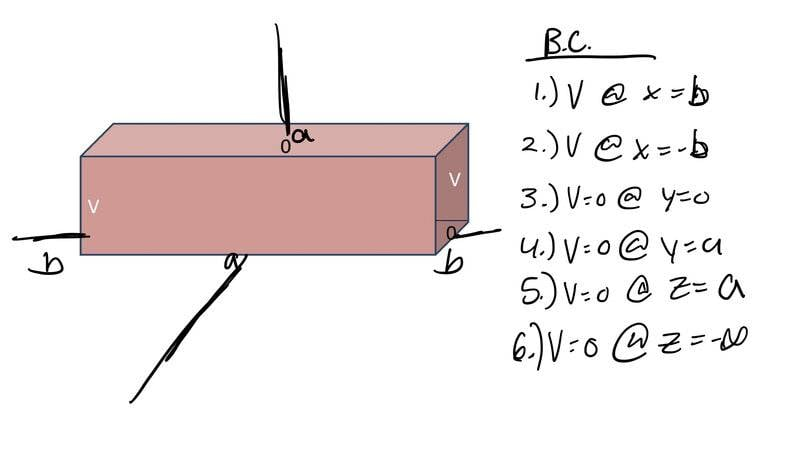# Boundary Conditions for an infinite rectangular pipe

guyvsdcsniper
Homework Statement:
An infinite rectangular pipe with sides a, has two opposite sides at voltage V
(front and back) and at voltage V=0 (top and bottom).

Find the potential inside the pipe.
Relevant Equations:
Fourier Sine Trick
Does setting up the problem symmetrically on this axis and the boundary conditions applied make sense? I don't believe I will have a problem solving for the potential inside, but i just want to make sure I have my B.C and axis correct before proceeding.EDIT:

Or should this be a 2-D lapace equation since the pipe is infinitely long, making this independent of the z axis?

Last edited:

Homework Helper
Gold Member
2022 Award
I'm a bit confused about how you are assigning the axes.
I'll call the left right axis X, the vertical axis Z and the lower left/ upper right axis in the picture Y. From that and the text description, I would say the pipe is infinite in the X axis and width a in the other two. The voltage is V on the faces normal to the Y axis and 0 on those normal to the Z axis.

guyvsdcsniper
I'm a bit confused about how you are assigning the axes.
I'll call the left right axis X, the vertical axis Z and the lower left/ upper right axis in the picture Y. From that and the text description, I would say the pipe is infinite in the X axis and width a in the other two. The voltage is V on the faces normal to the Y axis and 0 on those normal to the Z axis.
Sorry I missed that. y is the vertical axis.z is the axis coming out of the page. X is horizontal.

But I think it should be independent of Z since it is infinitely long. It mirrors an example straight out my textbook.